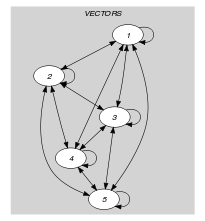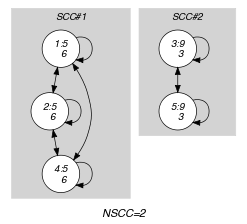## 5.291. nvectors

Origin
Constraint

$\mathrm{𝚗𝚟𝚎𝚌𝚝𝚘𝚛𝚜}\left(\mathrm{𝚅𝙴𝙲𝚃𝙾𝚁𝚂},\mathrm{𝚁𝙴𝙻𝙾𝙿},\mathrm{𝙻𝙸𝙼𝙸𝚃}\right)$

Synonym

$\mathrm{𝚗𝚙𝚘𝚒𝚗𝚝𝚜}$.

Type
 $\mathrm{𝚅𝙴𝙲𝚃𝙾𝚁}$ $\mathrm{𝚌𝚘𝚕𝚕𝚎𝚌𝚝𝚒𝚘𝚗}\left(\mathrm{𝚟𝚊𝚛}-\mathrm{𝚍𝚟𝚊𝚛}\right)$
Arguments
 $\mathrm{𝚅𝙴𝙲𝚃𝙾𝚁𝚂}$ $\mathrm{𝚌𝚘𝚕𝚕𝚎𝚌𝚝𝚒𝚘𝚗}\left(\mathrm{𝚟𝚎𝚌}-\mathrm{𝚅𝙴𝙲𝚃𝙾𝚁}\right)$ $\mathrm{𝚁𝙴𝙻𝙾𝙿}$ $\mathrm{𝚊𝚝𝚘𝚖}$ $\mathrm{𝙻𝙸𝙼𝙸𝚃}$ $\mathrm{𝚍𝚟𝚊𝚛}$
Restrictions
 $|\mathrm{𝚅𝙴𝙲𝚃𝙾𝚁}|\ge 1$ $\mathrm{𝚛𝚎𝚚𝚞𝚒𝚛𝚎𝚍}$$\left(\mathrm{𝚅𝙴𝙲𝚃𝙾𝚁𝚂},\mathrm{𝚟𝚎𝚌}\right)$ $\mathrm{𝚜𝚊𝚖𝚎}_\mathrm{𝚜𝚒𝚣𝚎}$$\left(\mathrm{𝚅𝙴𝙲𝚃𝙾𝚁𝚂},\mathrm{𝚟𝚎𝚌}\right)$ $\mathrm{𝚁𝙴𝙻𝙾𝙿}\in \left[=,\ne ,<,\ge ,>,\le \right]$
Purpose

Let $N$ be the number of distinct tuples of values taken by the vectors of the $\mathrm{𝚅𝙴𝙲𝚃𝙾𝚁𝚂}$ collection. Enforce condition $N\mathrm{𝚁𝙴𝙻𝙾𝙿}\mathrm{𝙻𝙸𝙼𝙸𝚃}$ to hold.

Example
$\left(\begin{array}{c}〈\begin{array}{c}\mathrm{𝚟𝚎𝚌}-〈5,6〉,\hfill \\ \mathrm{𝚟𝚎𝚌}-〈5,6〉,\hfill \\ \mathrm{𝚟𝚎𝚌}-〈9,3〉,\hfill \\ \mathrm{𝚟𝚎𝚌}-〈5,6〉,\hfill \\ \mathrm{𝚟𝚎𝚌}-〈9,3〉\hfill \end{array}〉,=,2\hfill \end{array}\right)$

The $\mathrm{𝚗𝚟𝚎𝚌𝚝𝚘𝚛𝚜}$ constraint holds since the number of distinct tuples of values (i.e., tuples $〈5,6〉$ and $〈9,3〉$) occurring within the collection $\mathrm{𝚅𝙴𝙲𝚃𝙾𝚁𝚂}$ is equal (i.e., $\mathrm{𝚁𝙴𝙻𝙾𝙿}$ is set to $=$) to its third argument $\mathrm{𝙻𝙸𝙼𝙸𝚃}=2$.

Typical
 $|\mathrm{𝚅𝙴𝙲𝚃𝙾𝚁}|>1$ $|\mathrm{𝚅𝙴𝙲𝚃𝙾𝚁𝚂}|>1$ $\mathrm{𝚁𝙴𝙻𝙾𝙿}\in \left[=,<,\ge ,>,\le \right]$ $\mathrm{𝙻𝙸𝙼𝙸𝚃}>1$ $\mathrm{𝙻𝙸𝙼𝙸𝚃}<|\mathrm{𝚅𝙴𝙲𝚃𝙾𝚁𝚂}|$
Symmetries
• Items of $\mathrm{𝚅𝙴𝙲𝚃𝙾𝚁𝚂}$ are permutable.

• Items of $\mathrm{𝚅𝙴𝙲𝚃𝙾𝚁𝚂}.\mathrm{𝚟𝚎𝚌}$ are permutable (same permutation used).

• All occurrences of two distinct values of $\mathrm{𝚅𝙴𝙲𝚃𝙾𝚁𝚂}.\mathrm{𝚟𝚎𝚌}$ can be swapped; all occurrences of a value of $\mathrm{𝚅𝙴𝙲𝚃𝙾𝚁𝚂}.\mathrm{𝚟𝚎𝚌}$ can be renamed to any unused value.

Arg. properties
• Contractible wrt. $\mathrm{𝚅𝙴𝙲𝚃𝙾𝚁𝚂}$ when $\mathrm{𝚁𝙴𝙻𝙾𝙿}\in \left[<,\le \right]$.

• Extensible wrt. $\mathrm{𝚅𝙴𝙲𝚃𝙾𝚁𝚂}$ when $\mathrm{𝚁𝙴𝙻𝙾𝙿}\in \left[\ge ,>\right]$.

Reformulation

The $\mathrm{𝚗𝚟𝚎𝚌𝚝𝚘𝚛𝚜}$$\left(\mathrm{𝚅𝙴𝙲𝚃𝙾𝚁𝚂},\mathrm{𝚁𝙴𝙻𝙾𝙿},\mathrm{𝙻𝙸𝙼𝙸𝚃}\right)$ constraint can be expressed in term of the conjunction $\mathrm{𝚗𝚟𝚎𝚌𝚝𝚘𝚛}$$\left(\mathrm{𝑁𝑉},\mathrm{𝚅𝙴𝙲𝚃𝙾𝚁𝚂}\right)$ $\wedge$ $\mathrm{𝑁𝑉}\mathrm{𝚁𝙴𝙻𝙾𝙿}\mathrm{𝙻𝙸𝙼𝙸𝚃}$.

specialisation: $\mathrm{𝚗𝚟𝚎𝚌𝚝𝚘𝚛}$ (replace a comparison with the number of distinct vectors by an equality with the number of distinct vectors).

Keywords
Arc input(s)

$\mathrm{𝚅𝙴𝙲𝚃𝙾𝚁𝚂}$

Arc generator
$\mathrm{𝐶𝐿𝐼𝑄𝑈𝐸}$$↦\mathrm{𝚌𝚘𝚕𝚕𝚎𝚌𝚝𝚒𝚘𝚗}\left(\mathrm{𝚟𝚎𝚌𝚝𝚘𝚛𝚜}\mathtt{1},\mathrm{𝚟𝚎𝚌𝚝𝚘𝚛𝚜}\mathtt{2}\right)$

Arc arity
Arc constraint(s)
$\mathrm{𝚕𝚎𝚡}_\mathrm{𝚎𝚚𝚞𝚊𝚕}$$\left(\mathrm{𝚟𝚎𝚌𝚝𝚘𝚛𝚜}\mathtt{1}.\mathrm{𝚟𝚎𝚌},\mathrm{𝚟𝚎𝚌𝚝𝚘𝚛𝚜}\mathtt{2}.\mathrm{𝚟𝚎𝚌}\right)$
Graph property(ies)
$\mathrm{𝐍𝐒𝐂𝐂}$$\mathrm{𝚁𝙴𝙻𝙾𝙿}\mathrm{𝙻𝙸𝙼𝙸𝚃}$

Graph class
$\mathrm{𝙴𝚀𝚄𝙸𝚅𝙰𝙻𝙴𝙽𝙲𝙴}$

Graph model

Parts (A) and (B) of Figure 5.291.1 respectively show the initial and final graph associated with the Example slot. Since we use the $\mathrm{𝐍𝐒𝐂𝐂}$ graph property we show the different strongly connected components of the final graph. Each strongly connected component corresponds to a tuple of values that is assigned to some vectors of the $\mathrm{𝚅𝙴𝙲𝚃𝙾𝚁𝚂}$ collection. The 2 following tuple of values $〈5,6〉$ and $〈9,3〉$ are used by the vectors of the $\mathrm{𝚅𝙴𝙲𝚃𝙾𝚁𝚂}$ collection.

##### Figure 5.291.1. Initial and final graph of the $\mathrm{𝚗𝚟𝚎𝚌𝚝𝚘𝚛𝚜}$ constraint(a) (b)